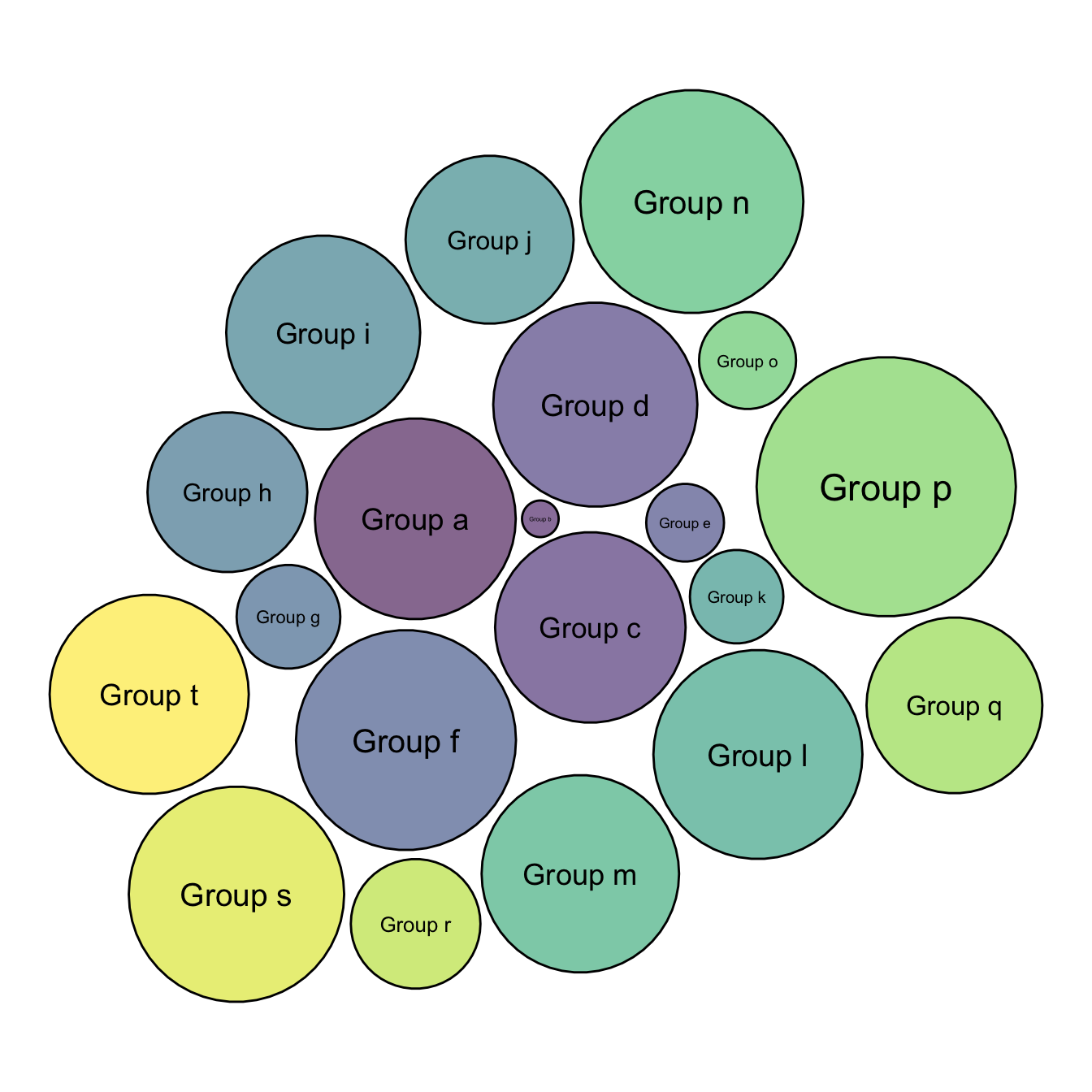# Circle packing customization with R

This posts explains how to build a one level circle packing chart with space between bubbles, improving the general appearance.

This chart is just a customisation of the chart #305 which describes the basic process to make a one level circle packing chart. I personally like to add a bit of space between each circle.

Basically, all you have to do is to reduce the `radius` size in your data once this one has been calculated. Just multiply it by a number under 0, and it will decrease the circle size.

If you have been so far, you probably want to check the interactive version of the chart !``````# libraries
library(packcircles)
library(ggplot2)
library(viridis)

# Create data
data <- data.frame(group=paste("Group", letters[1:20]), value=sample(seq(1,100),20))

# Generate the layout
packing <- circleProgressiveLayout(data\$value, sizetype='area')
data <- cbind(data, packing)
dat.gg <- circleLayoutVertices(packing, npoints=50)

# Plot
ggplot() +
geom_polygon(data = dat.gg, aes(x, y, group = id, fill=id), colour = "black", alpha = 0.6) +
scale_fill_viridis() +
geom_text(data = data, aes(x, y, size=value, label = group), color="black") +
theme_void() +
theme(legend.position="none")+
coord_equal()``````

Related chart types

## Contact

This document is a work by Yan Holtz. Any feedback is highly encouraged. You can fill an issue on Github, drop me a message on Twitter, or send an email pasting yan.holtz.data with gmail.com.